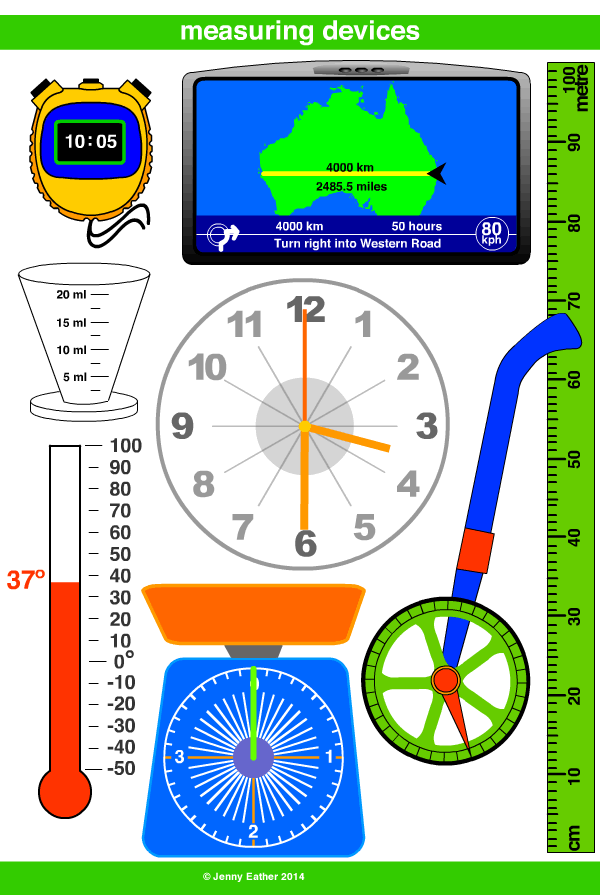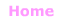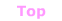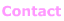Aa Bb Cc Dd Ee Ff Gg Hh Ii Jj Kk Ll Mm Nn Oo Pp Qq Rr Ss Tt Uu Vv Ww Xx Yy Zz

Mm

measure, measuring

• use of standard units to determine size or quantity
in regard to length, breadth, height, area,
mass or weight, volume, fluid volume,
capacity, temperature and time.

EXAMPLES:Metric Units ~ Length

10 millimetres = 1 centimetre, cm
10 centimetres = 1 decimetre, dm
10 decimetres = 1 metre, m
10 metres = 1 decametre, Dm or dkm
10 decametres = 1 hectometre, hm
10 hectometres = 1 kilometre, km
1000 metres = 1 kilometre, km

Metric Units ~ Area

1 square metre = 10 000 square centimetres, cm2
1 hectare = 10 000 square metres, m2
1 square kilometre = 100 hectares, ha

Metric Units ~ Mass

1000 milligrams = 1 gram, g
1000 grams = 1 kilogram, kg
1000 kilograms = 1 tonne, t

Metric Units ~ Volume

1000 cubic millimetres = 1 cubic centimetre, cm3
1000 cubic centimetres = 1 cubic decimetre, dm3
1,000 cubic decimetres = 1 cubic metre, m3
1,000,000 cubic centimetres = 1 cubic metre, m3

Metric Units ~ Capacity
(fluid volume)

10 millilitres = 1 centilitre, cl or cL
10 centilitres = 1 decilitre, dl or dL
10 decilitres = 1 litre, l or L
1000 litres = 1 kilolitre, kl or kL
1,000,000 litres = 1 megalitre, Ml or ML
1,000,000,000 litres = 1 gigalitre, Gl or GL
1,000,000,000,000 litres = cubic kilometre = 1 teralitre, Tl or TL

Metric Units ~ Temperature

Celsius, ºC also known as Centigrade, ºC

Imperial/Customary Units ~ Length

12 inches = 1 foot
3 feet = 1 yard
22 yards = 1 chain
10 chains = 1 furlong
8 furlongs = 1 mile
5280 feet = 1 mile
1760 yards = 1 mile

Imperial/Customary Units ~ Area

144 square inches = 1 square foot
9 square feet = 1 square yard
4,840 square yards = 1 acre
640 acres = 1 square mile

Imperial/Customary Units ~ Volume

1728 cubic inches = 1 cubic foot
27 cubic feet = 1 cubic yard

Imperial/Customary Units ~ Fluid Volume

8 fluid ounces = 1 cup (US)
10 fluid ounces = 1 cup (UK)
16 fluid ounces = 1 pint (US)
20 fluid ounces = 1 pint (UK)
2 pints = 1 quart
4 quarts = 1 gallon

1 gallon = 4 quarts, 8 pints, 16 cups

Imperial/Customary Units ~ Weight, Mass

437.5 grains = 1 ounce
16 ounces = 1 pound
14 pounds = 1 stone
8 stones = 1 hundredweight (UK)
100 pounds = 1 hundredweight (US)
20 hundredweight = 1 ton
1 ton = 2240 pounds (UK)
1 ton = 2000 pounds (US)

Imperial/Customary Units ~ Temperature

Fahrenheit ºF© Jenny Eather 2014. All rights reserved.